# Flight Radar : Building your own simple, real time Flight Monitor using Python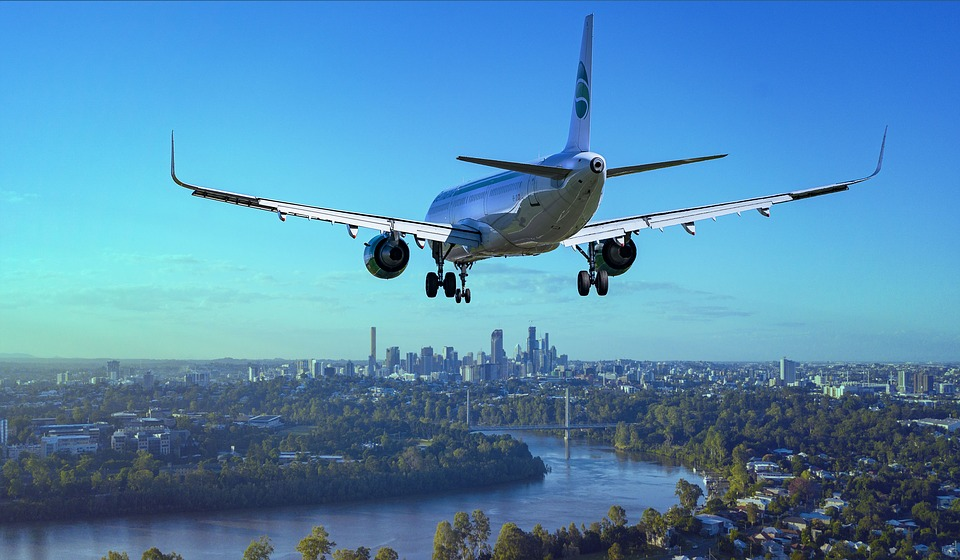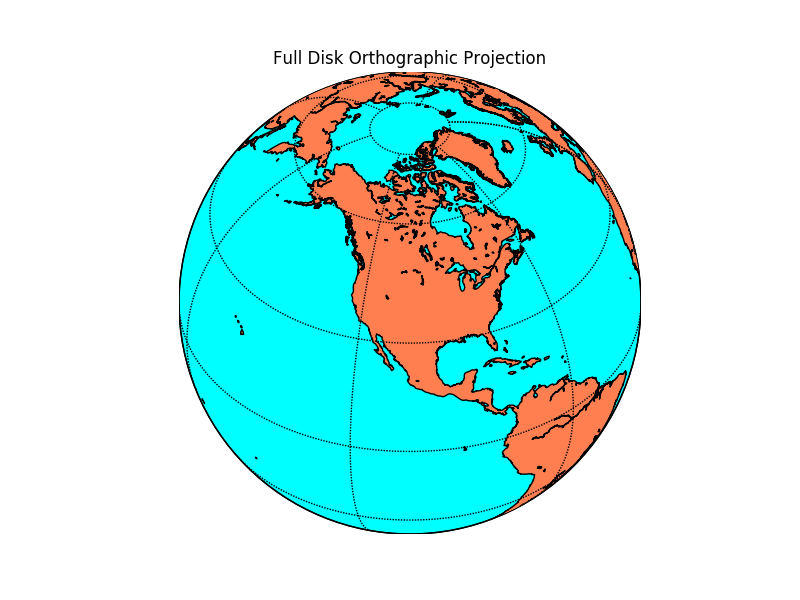# Introduction

According to a previous year report, there were around 9728 planes – carrying about 1,270,406 people – in the sky at a given moment! How are all the flights controlled and avoided from colliding with each other? Well, there are complex systems which monitor real-time sky traffic and provide necessary details to the pilot. In case the projectiles of two or more flights meet, a warning system is activated and the necessary plan to escape the collision is provided.

The aim of this article is not to inform you about ATC processes, however, we would be using the data from the servers of ICAO to make our own simplistic Flight Radar.  Every aircraft in air is assigned its own ICAO 24-bit address. This unique address of an aircraft is then used to access some important information like the location (latitude, longitude), flight altitude and velocity.

# Pre Requisites

1. Basic knowledge of Python and handling of Jupyter Notebooks.
2. Python, pip, and git installed on your machine.
3. Working with the terminal.
4. Libraries – Matplotlib, Basemap

If new to these points I advise you to look out for the Anaconda distribution. If you are on Windows, working with Anaconda Prompt is the best and easiest way to deal with development processes in python.

The basic requirement of our code is to fetch information from the ICAO 24 – bit address of each aircraft we care about. Well, there is a very simple and effective tool available for this. The Open Sky API! Just a call to this API will help us fetch the details of the aircraft we are looking for! 90% of the work done right?! That’s why I love APIs.

# Installing required packages

Now I advise you to make a separate folder, where you’ll work.

## MatplotlibIf you have Anaconda distribution you’ll be already having matplotlib installed. Matplotlib is a powerful Python library for fantastic data visualizations. If you are interested and passionate about visualizations I advice you to take a separate tutorial on matplotlib (and seaborn).

If you don’t have anaconda installed, you can fetch the package from the Python Package Index (PyPI).

Just run the following in your terminal

`pip install matplotlib`

## Matplotlib – Basemap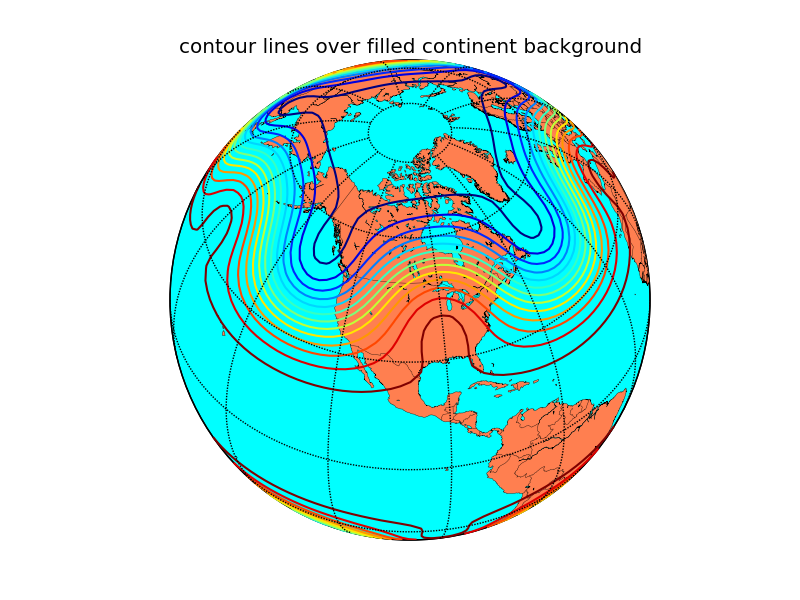Now we need Basemap. Basemap was developed by the same developers who had worked on matplotlib. Those were the years when python was being shaped into a multi-functional, easy to use, research-oriented language it is now. Ahh, the golden years of python!

Noo! Let’s not deviate!

Basemap offers easy integration with matplotlib, a very necessary function for our code to work. There are many other GIS specific great libraries in python but since we need integration with matplotlib ( and basemap is the only one I’ve worked with:) ) we will go with basemap. If you’re having conda it’s easy.

`conda install -c anaconda basemap`

If you do not have conda you need to clone the basemap github repository and then run setup.py

`git clone https://github.com/matplotlib/basemap.git`

Move to the directory where the repo is cloned and run

`python setup.py install`

## Open-Sky Network API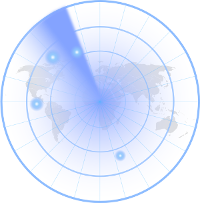The API is not available on either conda cloud or PyPI and so it requires manual installation.

```git clone https://github.com/openskynetwork/opensky-api.git
cd opensky-api/python
python setup.py install```

## High-Resolution Maps : Basemap (Optional)

By default, basemap has very minimalistic maps installed with it. However, if we really care about the deep details of our maps we should separately install high resolution maps. However this means our code will take more time to process since it requires to render such high detailed maps.

# Let’s Code!Now let’s open up our Jupyter Notebooks and import the required packages. In the same directory where you’ve installed OpenSky API, open jupyter notebook by simply typing in

`jupyter notebook`

## Importing requirements

```import matplotlib.pyplot as plt
from opensky_api import OpenSkyApi
from mpl_toolkits.basemap import Basemap
from IPython import display```

All the plots made by matplotlib are stored at a specific memory location while the code runs. When we run the code, it by default prints a message telling us where the plot is saved. However, if instead, we want all the plots to be displayed as soon as they are made inside our notebook we need to inform it explicitly.

`%matplotlib inline`

Now let’s define a function which will help us fetch the latitude and longitude of the flights (called states in OpenSky API documentation). Now by default, the OpenSky API returns the states of all the flights in contact with the ICAO servers. We do not want the details of all the flights in the sky. What we will do is define a box using latitude, longitude coordinates of its corners and fetch the data of only that box. This box will be over Indian Airspace. Hence we will fetch the details of flights only over Indian Airspace. Since it is a large area we will display the real-time movements of flights only in the region south of Tropic Of Cancer, which has some of the important Indian airports and also hosts many international flight routes.

The latitude, longitude coordinates can be easily known from Google Maps by clicking at random points within our desired box.

Let’s define a function which returns the latitude, longitude coordinates of the flights in the box specified.

```def coordinates():
api = OpenSkyApi()
lon = []
lat = []
j = 0
# bbox = (min latitude, max latitude, min longitude, max longitude)
states = api.get_states(bbox=(8.27, 33.074, 68.4, 95.63))
for s in states.states:
lon.append([])
lon[j] = s.longitude
lat.append([])
lat[j] = s.latitude
j+=1
return(lon, lat)
```

Here  api.get_states(…) helps us define the box under which we need to fetch the flights’ data. Here the bbox covers Indian Airspace. This code snippet is already commented and so it’s not difficult to understand. The loop iterates over all the states fetched from the bbox specified and we extract only the latitude and longitude out of it. At last, the (lat, lon) lists are returned which now possess the location of every flight over the Indian Airspace at the time you are reading this!

Now let’s plot the coordinates fetched on the Indian Airspace. As mentioned, to properly visualize the aircrafts’ movements over space we would consider only the region of Indian Airspace below the Tropic of Cancer.

What we will do here is plot the map with the coordinates on it and then re-plot for a certain number of times. Every time a new plot is displayed, coordinates of flights are shown for the time when the API was called. Since there is some inherent time taken by the code to print the high-resolution map, the next time we fetch the data from the API, we receive updated coordinates. This will help us show the exact path of each aircraft.

To display the plots one after the other it’s obvious we will use an iteration and plot the map for every iteration. Let’s also ask the user the number of iterations he/she wants to make it a bit interactive.

```print("How many Iterations?")
a = int(input())```

Now let’s code the iteration.

```for i in range(1, a + 1) :
fig_size = plt.rcParams["figure.figsize"]
fig_size = 20
fig_size = 20
plt.rcParams["figure.figsize"] = fig_size
lon, lat = coordinates()
m = Basemap(projection = 'mill', llcrnrlat = 8.1957,   urcrnrlat = 23.079, llcrnrlon = 68.933, urcrnrlon = 88.586, resolution = 'h')
m.drawcoastlines()
m.drawmapboundary(fill_color = '#FFFFFF')
x, y = m(lon, lat)
plt.scatter(x, y, s = 5)
display.clear_output(wait=True)
display.display(plt.gcf())```

Let’s break down and understand this for – loop step by step

```fig_size = plt.rcParams["figure.figsize"]
fig_size = 20
fig_size = 20
plt.rcParams["figure.figsize"] = fig_size```

What this chunk of code does is set a size to the plot image displayed in the Jupyter Notebook. By default the size is insufficient for us to monitor all the flights.

`lon, lat = coordinates()`

This is pretty obvious. coordinates function is called and the lists with the values of flight latitudes and longitudes are stored in the lists lat and lon respectively.

`m = Basemap(projection = 'mill', llcrnrlat = 8.1957,   urcrnrlat = 23.079, llcrnrlon = 68.933, urcrnrlon = 88.586, resolution = 'h')`

This code segment creates a basic Basemap. In cartography, there are many projections available to choose from. The miller projection is the simplest form of flat maps that we generally see. If you want to read more about map projections you can do it here.

llcrnrlat is just an abbreviation to lower left corner latitude. What these 4 variables do is again define a region for which the map has to be generated. In this case, it is the Southern part of India. I have set the resolution to high (‘h’) to render really high-quality maps. If you think it uses a lot of compute you can switch to lower quality by setting resolution = ‘c’ instead.

```m.drawcoastlines()
m.drawmapboundary(fill_color = '#FFFFFF')```

I think this is pretty readable. It draws coastlines and boundary for the map and sets map color to white.

```x, y = m(lon, lat)
plt.scatter(x, y, s = 5)```

This segment at last prints the coordinates on the basemap. plt.scatter(…) is actually a matplotlib function. This is where the integration comes into the picture which we had discussed in the introduction paragraph. Both the basemaps and scatterplot we created are plotted on single axes providing us with the final map!

```display.clear_output(wait=True)
display.display(plt.gcf())```

As soon as the plot is generated and printed the next time a plot is generated we need to remove the previous plot and display the present one. This code does exactly the same thing and hence adds “motion“ to the flights plotted!

## This is how the final plot looks like:

(look closely, this is a GIF which is exactly how your actual output will look like)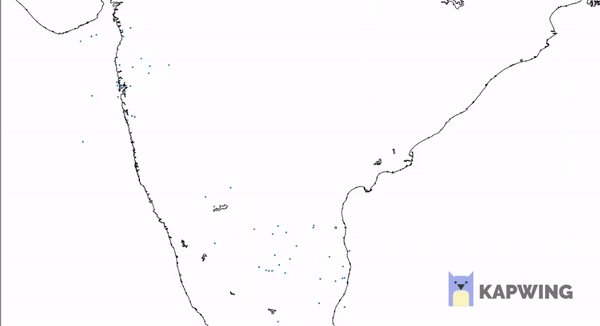CEV - Handout